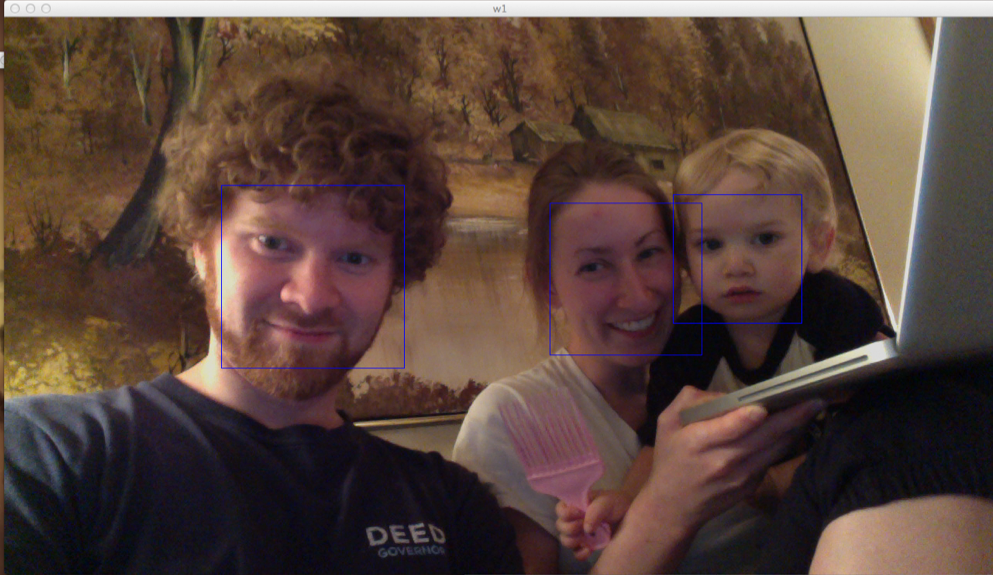# Facial Detection with OpenCV and Python

I was able to get some basic facial detection working in OpenCV with Python. Here's what it looks like:And here is the 34-line python script to draw boxes around all detected faces in live video:

import cv

CAMERA_INDEX = 0

def detect_faces(image):
faces = []
detected = cv.HaarDetectObjects(image, cascade, storage, 1.2, 2, cv.CV_HAAR_DO_CANNY_PRUNING, (100,100))
if detected:
for (x,y,w,h),n in detected:
faces.append((x,y,w,h))
return faces

if __name__ == "__main__":
cv.NamedWindow("Video", cv.CV_WINDOW_AUTOSIZE)

capture = cv.CaptureFromCAM(CAMERA_INDEX)
storage = cv.CreateMemStorage()
faces = []

i = 0
while True:
image = cv.QueryFrame(capture)

# Only run the Detection algorithm every 5 frames to improve performance
if i%5==0:
faces ...

# Intro to OpenCV with Python

I have started my journey into the world of OpenCV using Python on my Mac. These are my first steps with it.

# Installing OpenCV for Python on the Mac

# Install numpy as a prerequisite
sudo port install numpy

# Install OpenCV with the Python API
sudo port install opencv +python27


(The port command is part of Macports)

# Capturing an images from a webcam

  1 2 3 4 5 6 7 8 9 10 #!/usr/bin/env python2.7 import cv cv.NamedWindow("w1", cv.CV_WINDOW_AUTOSIZE) camera_index = 0 capture = cv.CaptureFromCAM(camera_index) frame = cv.QueryFrame(capture) cv.SaveImage("pic.jpg", frame) 

(I tested this with the iSight camera on my Macbook Pro)

# Displaying live video

  1 2 3 4 5 6 7 8 9 10 11 #!/usr/bin/env python2 ...

# Linear Regression with Gradient Descent

I'm taking the Stanford Machine Learning class. The first algorithm we covered is Linear Regression using Gradient Descent. I implemented this algorithm is Python. Here's what it looks like:

import subprocess

def create_hypothesis(slope, y0):
return lambda x: slope*x + y0

def linear_regression(data_points, learning_rate=0.01, variance=0.001):
""" Takes a set of data points in the form: [(1,1), (2,2), ...] and outputs (slope, y0). """

slope_guess = 1.
y0_guess = 1.

last_slope = 1000.
last_y0 = 1000.
num_points = len(data_points)

while (abs(slope_guess-last_slope) > variance or abs(y0_guess - last_y0) > variance):
last_slope = slope_guess
last_y0 = y0_guess

hypothesis = create_hypothesis(slope_guess, y0_guess)

y0_guess = y0_guess - learning_rate * (1./num_points) * sum([hypothesis(point) - point for point in data_points])
slope_guess = slope_guess - learning_rate * (1./num_points) * sum([ (hypothesis(point) - point) * point for point ...

# Resampling Wheel Algorithm

I've been taking the Udacity CS373 (Robotic Car) class, and this week the topic was Particle filters. Particle filters are basically a localization algorithm that accounts for error in measurements and sensors.

Anyway, part of the Particle Filter algorithm requires the generation of a new set of these things called "particles" based on the particles' weights. So to accomplish this task, the Resample Wheel algorithm was presented in class. It is a particularly elegant method of generating a new set of particles by randomly drawing from an old set of particles (with replacement). The particle weight determines the likelihood of it being picked.

Here is the algorithm (with print statements - I used these to help me understand how the algorithm works):

import random

def generate_new_particles(old_particles, weights ...